## LetsPlayMaths.Com

WELCOME TO THE WORLD OF MATHEMATICS

# Class-8 Linear Inequalities In One Variable

Introduction

Replacement Set

Solution Set

Properties of Inequalities

Steps to Solve Linear Inequalities in One Variable

Graphical Representation of the Solution of Linear Inequations

Linear Inequalities Worksheet

## Introduction

Statements like (x > 5, x + 5 < 8, 5x − 1 ≥ 3, (x+2)3 > 2x + 1) are known as Linear inequalities of one variable.
Linear inequalities in x variable can be written as:

px + q > 0

px + q < 0

px + q ≤ 0

px + q ≥ 0

Here, p and q are rational number and p ≠ 0.

Before we are doing sums of linear inequalities, we should know about Replacement Set and Solution Set.

## Replacement Set

The set from which the value of variable in the inequality are chosen is known as replacement set.

## Solution Set

A solution of an inequality is a number which are chosen from the replacement set which, when substituted for the variable, that make the inequality true. The set of all the solutions of an inequality is called the solution set or truth set of the inequality.

Example. Let's consider the inequality x < 6 and replacement set is {1, 2, 3, 4, 5, 6, 7, 8, 9}.
Here, the value of x must be less than 6 and chosen from replacement set and make new set called solution set {1, 2, 3, 4, 5}.

Note:- Solution set always depend upon Replacement set.

Table for the inequality x < 9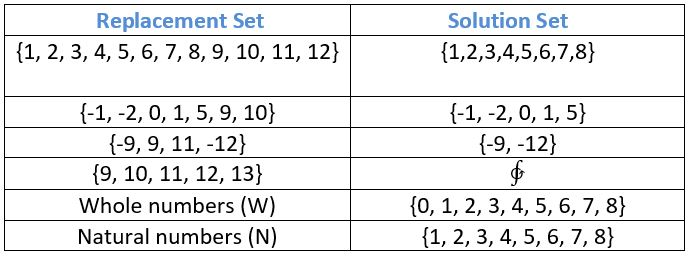## Properties of Inequalities

a) Adding the same expression or number to both side of an inequality, does not change the inequality.

If p > q, then p + r > q + r
If p < q, then p + r < q + r

Example 1. On adding 7 to each side of 14 > 9, does not change the inequality

Solution. 14 > 9 ⇒ (14 + 7) > (9 + 7)

⇒ 21 > 16

b) Subtracting the same expression or number from both side of an inequality, does not change the inequality.

If p > q, then p - r > q + r
If p < q, then p - r < q + r

Example. 2 On subtracting 5 from each side of 9 < 15, does not change the inequality

Solution. 9 < 15 ⇒ (9 − 5) < (15 − 5)

⇒ 4 < 10

c) Multiplying both side of an inequality by positive number, does not change the inequality.

If p > q, then p × r > q × r, here r > 0
If p < q, then p × r < q × r, here r > 0

Example 3. On multiplying each side of 15 > 6 by 2, does not change the inequality.

Solution. 15 > 6 ⇒ 15 × 2 > 6 × 2

⇒ 30 > 12

d) Multiplying each side of an inequality by a negative number, reverses the inequality.

If p > q, then p × r < q × r, here r < 0
If p < q, then p × r > q × r, here r < 0

Example 4. On multiplying each side of 5 < 9 by -3, reverses the inequality

Solution. 5 < 9 ⇒ 5 × (-3) < 9 × (-3)

⇒ -15 > -27

e) Dividing both side of an inequality by positive number, does not change the inequality.

If p > q, then p ÷ r > q ÷ r, here r > 0
If p < q, then p ÷ r < q ÷ r, here r > 0

Example 5. On dividing each side of 12 > 6 by 2, does not change the inequality.

Solution. 12 > 7 ⇒ (12 ÷ 2) > (6 ÷ 2)

⇒ 6 > 3

f) Dividing each side of an inequality by a negative number, reverses the inequality.

If p > q, then p ÷ r < q ÷ r, here r < 0
If p < q, then p ÷ r > q ÷ r, here r < 0

Example 6. On dividing each side of 27 > 18 by -9, reverses the inequality.

Solution. 27 > 18 ⇒ 27 ÷ (-9) > 18 ÷ (-9)

⇒ -3 < -2

## Steps to Solve Linear Inequalities in One Variable

a) Simplify both side by removing group symbols and collecting like terms.

b) Remove fractions or decimals by multiplying both sides by LCM of denominators or a power of 10 in case of decimals.

c) Write all variable term on one side and all constants on the other side. Then collect like terms.

d) Make the coefficient of the variable is 1.

e) Choose the solution set from the replacement set.

Note: We will be using following notation for Replacement set

N = Set of all natural numbers

W = set of all whole numbers

I = Set of all integers

Let's see some solved examples.

Example 1. Solve 4x + 2 ≤ 18, x ∈ N

Solution. Given 4x + 2 ≤ 18

⇒ 4x + 2 − 2 ≤ 18 − 2 (here subtracting 2 from both side)

⇒ 4x ≤ 16

⇒ 4x4164 (Dividing both sides by 4)

⇒ x ≤ 4

As x ∈ N, the solution set = {1, 2, 3, 4}

Example 2. Solve the inequality {(3p-1)5} ≤ 7, p ∈ W

Solution. Given {(3p-1)5} ≤ 7

Multiplying both the side by 5

⇒ 3p − 1 ≤ 35

⇒ 3p − 1 + 1 ≤ 35 +1 (adding 1 to both side)

⇒ 3p ≤ 36 (Dividing both side by 3)

⇒ p ≤ 12

As p ∈ W, the solution set = {0, 1, 2, 3, 4, 5, 6, 7, 8, 9, 10, 11, 12}

Example 3. Solve 12 + 5y > 7, where y is a negative integer.

Solution. 12 + 5y − 12 > 2 − 12

⇒ 5y > −10

⇒ Y > −2

As y is a negative integer, the solution set is {−1}.

Example 4. solve the inequality 5 − 2x ≥ x − 16, x ∈ W

Solution. 5 − 2x ≥ x − 16

⇒ -2x − x ≥ -16 − 5

⇒ -3x ≥ -21

⇒ -3x-3-21-3

⇒ x ≥ 7

Solution set of x is = {0, 1, 2, 3, 4, 5, 6, 7}

## Graphical Representation of the Solution of Linear Inequations

Solution of inequation can be represented on a number line. Let's see some examples.

Example 1. Represent the solution of m < 7, m ∈ N

Solution. Given m < 7, m ∈ N

The solution set = {1, 2, 3, 4, 5, 6}.

Then solution set can be represented by thick dot on the number line as shown below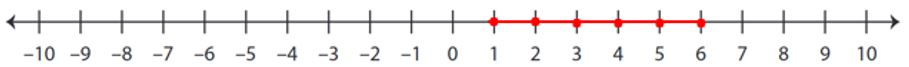Example 2. Represent the solution of -3 < x < 8, x ∈ I

Solution. Given -3 < x < 8, x ∈ I

The solution set = {-2, -1, 0, 1, 2, 3, 4, 5, 6, 7}

The solution set can be represented by thick dot on number line as shown below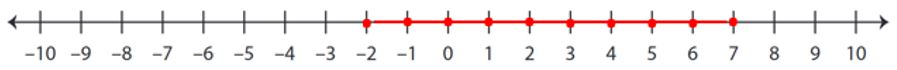Example 3. Solve the following inequation and show the solution set on a number line -1 ≤ x + 4 < 5

Solution. -1 ≤ x + 4 < 5

⇒ -1 ≤ x < 5 − 4

⇒ -1 ≤ x < 1

So, solution set of x = {0}

The solution set can be represented by thick dot on number line as shown below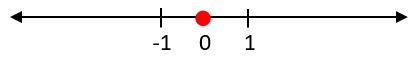Example 4. Given 3 − 4p < 9 − p, p ∈ I

Solution. 3 − 4p < 9 − p

⇒-4p + p < 9 -3

⇒ -3p < 6

⇒ -3p-3 < 6-3

⇒ p > -2

Solution set of p = {-1, 0, 1, 2, 3, ...}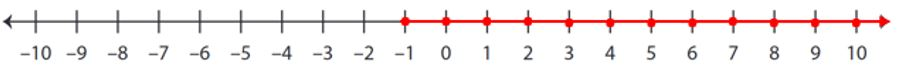The dots on right hand side show that the marking continues on right hand side up to infinity.

## Class-8 Linear Inequalities Worksheet

Linear Inequalities Worksheet - 1

Linear Inequalities Worksheet - 2